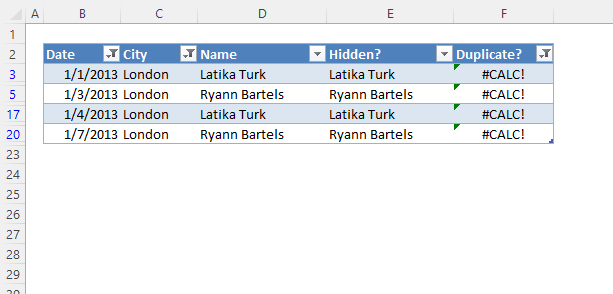Author: Oscar Cronquist Article last updated on October 13, 2022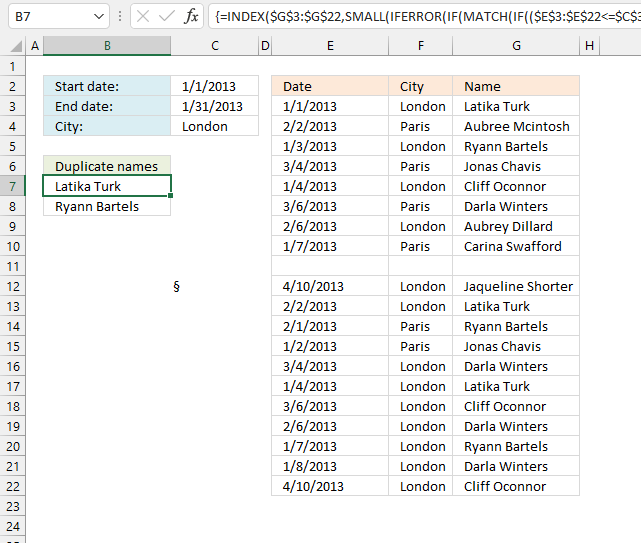This article demonstrates formulas and Excel tools that extract duplicates based on three conditions. The first and second condition is a date range, a start date, and an end date. The third condition is value.

Section 3 below demonstrates how to extract duplicates using the AutoFilter feature, this makes it even easier to apply whatever criteria you want.

## 1. Filter duplicate values using criteriaThe following worksheet allows you to search for duplicate names using a date range (cell B1 and B2) and a condition (cell B3).

The result is displayed in cells A6 and below.

Array formula in cell A6:

=INDEX(\$G\$3:\$G\$22, SMALL(IFERROR(IF(MATCH(IF((\$E\$3:\$E\$22<=\$C\$3)*(\$E\$3:\$E\$22>=\$C\$2)*(\$F\$3:\$F\$22=\$C\$4), \$G\$3:\$G\$22, ""), IF((\$E\$3:\$E\$22<=\$C\$3)*(\$E\$3:\$E\$22>=\$C\$2)*(\$F\$3:\$F\$22=\$C\$4), \$G\$3:\$G\$22, "A"), 0)<>MATCH(ROW(\$G\$3:\$G\$22), ROW(\$G\$3:\$G\$22)), MATCH(ROW(\$G\$3:\$G\$22), ROW(\$G\$3:\$G\$22))), ""), ROW(A1)))

### 1.1 How to enter an array formula

1. Copy (Ctrl + c) above formula.
2. Double press with the left mouse button on cell A6.
3. Paste (Ctrl + v) to cell A6.
4. Press and hold CTRL + SHIFT simultaneously.
5. Press Enter once.
6. Release all keys.

Your formula now looks like this: {=array_formula}
Don't enter the curly brackets, they appear automatically.

### 1.2 Explaining formula in cell A6

#### Step 1 - Compare end date to dates

The less than sign and the equal sign are logical operators, they let you check if the end date is larger than or equal to dates in cell range \$D\$2:\$D\$21.

The output is an array with the same size as the cell range, it contains boolean values TRUE or FALSE.

\$E\$2:\$E\$21<=\$C\$3

becomes

{41275; 41307; 41277; 41337; 41278; 41339; 41311; 41281; 0; 41374; 41307; 41306; 41276; 41337; 41278; 41339; 41311; 41281; 41282; 41374}<=41305

and returns

{TRUE; FALSE; TRUE; FALSE; TRUE; FALSE; FALSE; TRUE; TRUE; FALSE; FALSE; FALSE; TRUE; FALSE; TRUE; FALSE; FALSE; TRUE; TRUE; FALSE}.

#### Step 2 - Compare start date to dates

\$E\$2:\$E\$21>=\$C\$2

becomes

{41275; 41307; 41277; 41337; 41278; 41339; 41311; 41281; 0; 41374; 41307; 41306; 41276; 41337; 41278; 41339; 41311; 41281; 41282; 41374}<=41275

and returns

{TRUE; TRUE; TRUE; TRUE; TRUE; TRUE; TRUE; TRUE; FALSE; TRUE; TRUE; TRUE; TRUE; TRUE; TRUE; TRUE; TRUE; TRUE; TRUE; TRUE}

#### Step 3 - Check condition (city)

\$F\$3:\$F\$22=\$C\$4

becomes

{"London";"Paris";"London";"Paris";"London";"Paris";"London";"Paris";0;"London";"London";"Paris";"Paris";"London";"London";"London";"London";"London";"London";"London"}="London"

and returns

{TRUE; FALSE; TRUE; FALSE; TRUE; FALSE; TRUE; FALSE; FALSE; TRUE; TRUE; FALSE; FALSE; TRUE; TRUE; TRUE; TRUE; TRUE; TRUE; TRUE}.

#### Step 4 - Multiply arrays (AND Logic)

This step applies AND logic to the arrays. This means that both boolean values must be TRUE to return TRUE.

TRUE * TRUE = TRUE, FALSE* TRUE = FALSE, TRUE * FALSE= FALSE, FALSE* FALSE= FALSE.

(\$D\$2:\$D\$21<=\$B\$2)*(\$D\$2:\$D\$21>=\$B\$1)*(\$E\$2:\$E\$21=\$B\$3)

becomes

{TRUE; FALSE; TRUE; FALSE; TRUE; FALSE; FALSE; TRUE; TRUE; FALSE; FALSE; FALSE; TRUE; FALSE; TRUE; FALSE; FALSE; TRUE; TRUE; FALSE} * {TRUE; TRUE; TRUE; TRUE; TRUE; TRUE; TRUE; TRUE; FALSE; TRUE; TRUE; TRUE; TRUE; TRUE; TRUE; TRUE; TRUE; TRUE; TRUE; TRUE} * {TRUE; FALSE; TRUE; FALSE; TRUE; FALSE; TRUE; FALSE; FALSE; TRUE; TRUE; FALSE; FALSE; TRUE; TRUE; TRUE; TRUE; TRUE; TRUE; TRUE}

and returns

{1; 0; 1; 0; 1; 0; 0; 0; 0; 0; 0; 0; 0; 0; 1; 0; 0; 1; 1; 0}.

Note, boolean values are converted into their numerical equivalents when a calculation is made. TRUE = 1 and FALSE = 0 (zero).

#### Step 5 - Filter values in column F based on criteria

The IF function returns one value if the logical test is TRUE and another value if the logical test is FALSE.

Function syntax: IF(logical_test, [value_if_true], [value_if_false])

IF((\$E\$3:\$E\$22<=\$C\$3)*(\$E\$3:\$E\$22>=\$C\$2)*(\$F\$3:\$F\$22=\$C\$4), \$G\$3:\$G\$22, "")

becomes

IF({1; 0; 1; 0; 1; 0; 0; 0; 0; 0; 0; 0; 0; 0; 1; 0; 0; 1; 1; 0}, {"Latika Turk"; "Aubree Mcintosh"; "Ryann Bartels"; "Jonas Chavis"; "Cliff Oconnor"; "Darla Winters"; "Aubrey Dillard"; "Carina Swafford"; 0; "Jaqueline Shorter"; "Latika Turk"; "Ryann Bartels"; "Jonas Chavis"; "Darla Winters"; "Latika Turk"; "Cliff Oconnor"; "Darla Winters"; "Ryann Bartels"; "Darla Winters"; "Cliff Oconnor"}, "")

and returns

{"Latika Turk"; ""; "Ryann Bartels"; ""; "Cliff Oconnor"; ""; ""; ""; ""; ""; ""; ""; ""; ""; "Latika Turk"; ""; ""; "Ryann Bartels"; "Darla Winters"; ""}

#### Step 6 - Match filtered values

The MATCH function returns the relative position of an item in an array that matches a specified value in a specific order.

Function syntax: MATCH(lookup_value, lookup_array, [match_type])

MATCH(IF((\$E\$3:\$E\$22<=\$C\$3)*(\$E\$3:\$E\$22>=\$C\$2)*(\$F\$3:\$F\$22=\$C\$4), \$G\$3:\$G\$22, ""), IF((\$E\$3:\$E\$22<=\$C\$3)*(\$E\$3:\$E\$22>=\$C\$2)*(\$F\$3:\$F\$22=\$C\$4), \$G\$3:\$G\$22, "A"), 0)

becomes

MATCH({"Latika Turk"; ""; "Ryann Bartels"; ""; "Cliff Oconnor"; ""; ""; ""; ""; ""; ""; ""; ""; ""; "Latika Turk"; ""; ""; "Ryann Bartels"; "Darla Winters"; ""}, {"Latika Turk"; "A"; "Ryann Bartels"; "A"; "Cliff Oconnor"; "A"; "A"; "A"; "A"; "A"; "A"; "A"; "A"; "A"; "Latika Turk"; "A"; "A"; "Ryann Bartels"; "Darla Winters"; "A"}, 0)

and returns

{1; #N/A; 3; #N/A; 5; #N/A; #N/A; #N/A; #N/A; #N/A; #N/A; #N/A; #N/A; #N/A; 1; #N/A; #N/A; 3; 19; #N/A}.

#### Step 7 - Filter values based on being a duplicate

The ROW function calculates the row number of a cell reference.

Function syntax: ROW(reference)

IF(MATCH(IF((\$D\$2:\$D\$21<=\$B\$2)*(\$D\$2:\$D\$21>=\$B\$1)*(\$E\$2:\$E\$21=\$B\$3),\$F\$2:\$F\$21,""), IF((\$D\$2:\$D\$21<=\$B\$2)*(\$D\$2:\$D\$21>=\$B\$1)*(\$E\$2:\$E\$21=\$B\$3), \$F\$2:\$F\$21,"A"), 0)<>MATCH(ROW(\$F\$2:\$F\$21), ROW(\$F\$2:\$F\$21)), MATCH(ROW(\$F\$2:\$F\$21), ROW(\$F\$2:\$F\$21)))

becomes

IF(MATCH({1; #N/A; 3; #N/A; 5; #N/A; #N/A; #N/A; #N/A; #N/A; #N/A; #N/A; #N/A; #N/A; 1; #N/A; #N/A; 3; 19; #N/A}<>{1; 2; 3; 4; 5; 6; 7; 8; 9; 10; 11; 12; 13; 14; 15; 16; 17; 18; 19; 20}, {1; 2; 3; 4; 5; 6; 7; 8; 9; 10; 11; 12; 13; 14; 15; 16; 17; 18; 19; 20})

and returns

{FALSE; #N/A; FALSE; #N/A; FALSE; #N/A; #N/A; #N/A; #N/A; #N/A; #N/A; #N/A; #N/A; #N/A; 15; #N/A; #N/A; 18; FALSE; #N/A}.

#### Step 8 - Remove error values

The MATCH function returns the relative position of an item in an array that matches a specified value in a specific order.

Function syntax: MATCH(lookup_value, lookup_array, [match_type])

IFERROR({FALSE; #N/A; FALSE; #N/A; FALSE; #N/A; #N/A; #N/A; #N/A; #N/A; #N/A; #N/A; #N/A; #N/A; 15; #N/A; #N/A; 18; FALSE; #N/A}, "")

and returns

{FALSE; ""; FALSE; ""; FALSE; ""; ""; ""; ""; ""; ""; ""; ""; ""; 15; ""; ""; 18; FALSE; ""}.

#### Step 9 - Extract k-th smallest row number

The SMALL function returns the k-th smallest value from a group of numbers.

Function syntax: SMALL(array, k)

SMALL(IFERROR(IF(MATCH(IF((\$D\$2:\$D\$21<=\$B\$2)*(\$D\$2:\$D\$21>=\$B\$1)*(\$E\$2:\$E\$21=\$B\$3),\$F\$2:\$F\$21,""), IF((\$D\$2:\$D\$21<=\$B\$2)*(\$D\$2:\$D\$21>=\$B\$1)*(\$E\$2:\$E\$21=\$B\$3), \$F\$2:\$F\$21,"A"), 0)<>MATCH(ROW(\$F\$2:\$F\$21), ROW(\$F\$2:\$F\$21)), MATCH(ROW(\$F\$2:\$F\$21), ROW(\$F\$2:\$F\$21))), ""), ROW(A1))

becomes

SMALL({FALSE; ""; FALSE; ""; FALSE; ""; ""; ""; ""; ""; ""; ""; ""; ""; 15; ""; ""; 18; FALSE; ""}, ROW(A1))

becomes

SMALL({FALSE; ""; FALSE; ""; FALSE; ""; ""; ""; ""; ""; ""; ""; ""; ""; 15; ""; ""; 18; FALSE; ""}, 1)

and returns 15.

#### Step 10 - Get value based on a row number

The INDEX function returns a value or reference from a cell range or array, you specify which value based on a row and column number.

Function syntax: INDEX(array, [row_num], [column_num])

INDEX(\$F\$2:\$F\$21, SMALL(IFERROR(IF(MATCH(IF((\$D\$2:\$D\$21<=\$B\$2)*(\$D\$2:\$D\$21>=\$B\$1)*(\$E\$2:\$E\$21=\$B\$3),\$F\$2:\$F\$21,""), IF((\$D\$2:\$D\$21<=\$B\$2)*(\$D\$2:\$D\$21>=\$B\$1)*(\$E\$2:\$E\$21=\$B\$3), \$F\$2:\$F\$21,"A"), 0)<>MATCH(ROW(\$F\$2:\$F\$21), ROW(\$F\$2:\$F\$21)), MATCH(ROW(\$F\$2:\$F\$21), ROW(\$F\$2:\$F\$21))), ""), ROW(A1)))

becomes

INDEX(\$F\$2:\$F\$21, 15)

and returns

"Latika Turk".

## 2. Filter duplicate values using criteria - Excel 365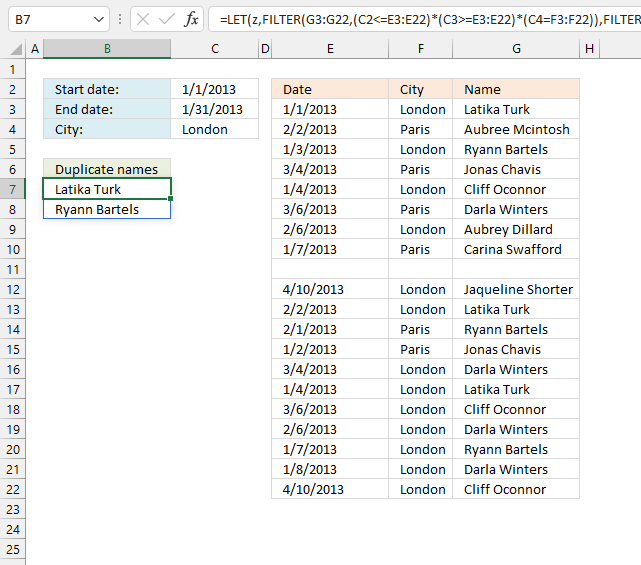Formula in cell B7:

=LET(z, FILTER(G3:G22, (C2<=E3:E22)*(C3>=E3:E22)*(C4=F3:F22)), FILTER(z, MATCH(z, z, 0)<>SEQUENCE(ROWS(z))))

### 2.1 Explaining formula

#### Step 1 - Compare dates to the start date

The less than character and the equal sign combined check if the dates are earlier or equal to the start date.

C2<=E3:E22

becomes

41275<={41275; 41307; 41277; 41337; 41278; 41339; 41311; 41281; 0; 41374; 41307; 41306; 41276; 41337; 41278; 41339; 41311; 41281; 41282; 41374}

and returns

{TRUE; TRUE; TRUE; TRUE; TRUE; TRUE; TRUE; TRUE; FALSE; TRUE; TRUE; TRUE; TRUE; TRUE; TRUE; TRUE; TRUE; TRUE; TRUE; TRUE}.

#### Step 2 - Compare dates to end date

The greater than character and the equal sign combined check if the dates are later or equal to the start date.

C3>=E3:E22

becomes

41305>={41275; 41307; 41277; 41337; 41278; 41339; 41311; 41281; 0; 41374; 41307; 41306; 41276; 41337; 41278; 41339; 41311; 41281; 41282; 41374}

and returns

{TRUE; FALSE; TRUE; FALSE; TRUE; FALSE; FALSE; TRUE; TRUE; FALSE; FALSE; FALSE; TRUE; FALSE; TRUE; FALSE; FALSE; TRUE; TRUE; FALSE}.

#### Step 3 - Check the condition

The equal sign checks if the condition is equal to values in cell range F3:F22.

C4=F3:F22

becomes

"London"={"London";"Paris";"London";"Paris";"London";"Paris";"London";"Paris";0;"London";"London";"Paris";"Paris";"London";"London";"London";"London";"London";"London";"London"}

and returns

{TRUE; FALSE; TRUE; FALSE; TRUE; FALSE; TRUE; FALSE; FALSE; TRUE; TRUE; FALSE; FALSE; TRUE; TRUE; TRUE; TRUE; TRUE; TRUE; TRUE}.

#### Step 4 - Multiply arrays (AND logic)

The asterisk allows you to multiply arrays. The arrays contain either TRUE or FALSE. Multiplying boolean values TRUE or FALSE creates AND - logic meaning both values must be TRUE to return TRUE.

In other words, all three conditions must be met in order to return TRUE.

(C2<=E3:E22)*(C3>=E3:E22)*(C4=F3:F22)

becomes

{TRUE; TRUE; TRUE; TRUE; TRUE; TRUE; TRUE; TRUE; FALSE; TRUE; TRUE; TRUE; TRUE; TRUE; TRUE; TRUE; TRUE; TRUE; TRUE; TRUE} * {TRUE; FALSE; TRUE; FALSE; TRUE; FALSE; FALSE; TRUE; TRUE; FALSE; FALSE; FALSE; TRUE; FALSE; TRUE; FALSE; FALSE; TRUE; TRUE; FALSE} * {TRUE; FALSE; TRUE; FALSE; TRUE; FALSE; TRUE; FALSE; FALSE; TRUE; TRUE; FALSE; FALSE; TRUE; TRUE; TRUE; TRUE; TRUE; TRUE; TRUE}

and returns

{1; 0; 1; 0; 1; 0; 0; 0; 0; 0; 0; 0; 0; 0; 1; 0; 0; 1; 1; 0}.

#### Step 5 - Filter values based on criteria

The FILTER function filters values in a given cell range based on a condition or criteria.

FILTER(G3:G22, (C2<=E3:E22)*(C3>=E3:E22)*(C4=F3:F22))

becomes

FILTER(G3:G22, {1; 0; 1; 0; 1; 0; 0; 0; 0; 0; 0; 0; 0; 0; 1; 0; 0; 1; 1; 0})

and returns

{"Latika Turk"; "Ryann Bartels"; "Cliff Oconnor"; "Latika Turk"; "Ryann Bartels"; "Darla Winters"}.

The LET function lets you name intermediate calculation results which can shorten formulas considerably and improve performance.

Function syntax: LET(name1, name_value1, calculation_or_name2, [name_value2, calculation_or_name3...])

z - FILTER(G3:G22, (C2<=E3:E22)*(C3>=E3:E22)*(C4=F3:F22))

#### Step 6 - Match filtered values

The MATCH function returns a number representing the relative position of an item in an array or cell range.

MATCH(z, z, 0)

becomes

MATCH({"Latika Turk"; "Ryann Bartels"; "Cliff Oconnor"; "Latika Turk"; "Ryann Bartels"; "Darla Winters"}, {"Latika Turk"; "Ryann Bartels"; "Cliff Oconnor"; "Latika Turk"; "Ryann Bartels"; "Darla Winters"}, 0)

and returns

{1; 2; 3; 1; 2; 6}.

#### Step 7 - Create a sequence based on the number of filtered values

The ROWS function calculate the number of rows in a cell range.

Function syntax: ROWS(array)

ROWS(z)

becomes

ROWS({"Latika Turk"; "Ryann Bartels"; "Cliff Oconnor"; "Latika Turk"; "Ryann Bartels"; "Darla Winters"})

and returns

6.

The SEQUENCE function returns a sequence of numbers.

SEQUENCE(rows, [columns], [start], [step])

SEQUENCE(ROWS(z))

becomes

SEQUENCE(6)

and returns

{1; 2; 3; 4; 5; 6}.

#### Step 8 - Check for duplicates

The less than and greater than characters return TRUE if the numbers don't match indicating that the value is a duplicate.

MATCH(z, z, 0)<>SEQUENCE(ROWS(z))

becomes

{1; 2; 3; 1; 2; 6}<>{1; 2; 3; 4; 5; 6}

and returns

{FALSE; FALSE; FALSE; TRUE; TRUE; FALSE}.

#### Step 9 - Filter duplicate values

The FILTER function extracts values/rows based on a condition or criteria.

Function syntax: FILTER(array, include, [if_empty])

FILTER(z, MATCH(z, z, 0)<>SEQUENCE(ROWS(z)))

becomes

FILTER({"Latika Turk"; "Ryann Bartels"; "Cliff Oconnor"; "Latika Turk"; "Ryann Bartels"; "Darla Winters"}, {FALSE; FALSE; FALSE; TRUE; TRUE; FALSE})

and returns

{"Latika Turk"; "Ryann Bartels"}.

## 3. Filter duplicate values using criteria - Autofilter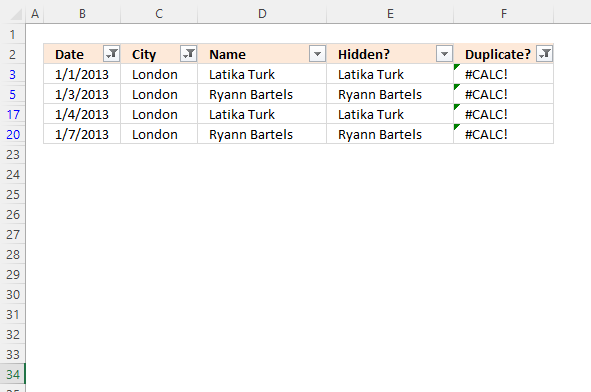This example shows how to filter duplicate values using the Autofilter and three conditions. Two conditions are needed to create a date range, start and end date. The third condition is the city.

I need two simple formulas to tell me if a name is visible (not filtered out) and a duplicate. The AutoFilter takes care of the conditions. Here is how:

### 3.1 Check if the value is hidden/filtered out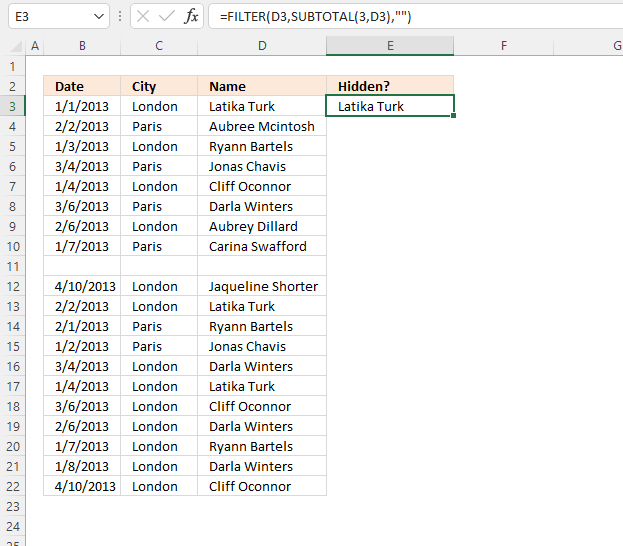This formula shows the value in the adjacent cell to the left if the value is not hidden or not empty.

Formula in cell E3:

=IF(SUBTOTAL(3,D3),D3,"")

Copy cell E3 and paste to cells below as far as needed.

### Explaining formula

#### Step 1 - Check if value is not empty and not hidden

The SUBTOTAL function returns a subtotal from a list or database, you can choose from a variety of arguments that determine what you want the function to do.

Function syntax: SUBTOTAL(function_num, ref1, ...)

SUBTOTAL(3,D3)

Argument 3 represents COUNTA meaning it counts not empty cells. Only cell reference D3 is used, COUNTA will return 1 or 0 (zero) which are the numerical equivalents to TRUE and FALSE respectively.

#### Step 2 - Show value if not empty and not hidden

The IF function returns one value if the logical test is TRUE and another value if the logical test is FALSE.

Function syntax: IF(logical_test, [value_if_true], [value_if_false])

IF(SUBTOTAL(3,D3),D3,"")

becomes

IF(1,"Latika Turk","")

and returns

"Latika Turk".

### 3.2 Check if the value is a duplicate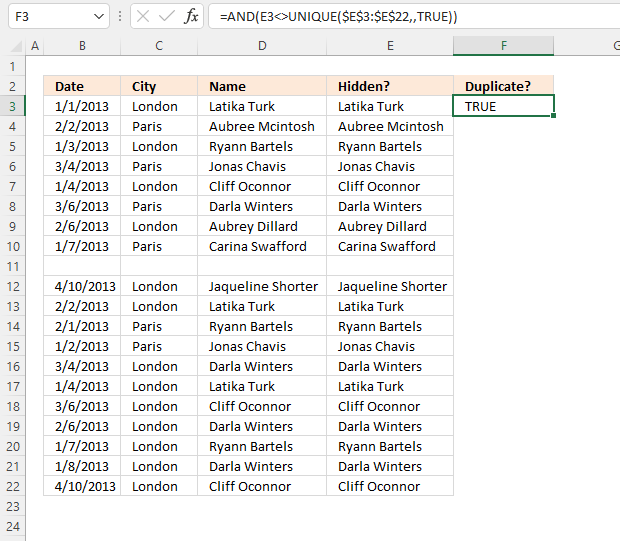Formula in cell F3:

=COUNTIF(\$E\$3:\$E\$22,E3)>1

Copy cell F3 and paste it to the cells below as far as needed.

### Explaining formula

#### Step 1 - Count values in cell range E3:E22 based on condition in cell E3

The COUNTIF function calculates the number of cells that is equal to a condition.

Function syntax: COUNTIF(range, criteria)

COUNTIF(\$E\$3:\$E\$22,E3)

becomes

=COUNTIF({"Latika Turk";"Aubree Mcintosh";"Ryann Bartels";"Jonas Chavis";"Cliff Oconnor";"Darla Winters";"Aubrey Dillard";"Carina Swafford";"";"Jaqueline Shorter";"Latika Turk";"Ryann Bartels";"Jonas Chavis";"Darla Winters";"Latika Turk";"Cliff Oconnor";"Darla Winters";"Ryann Bartels";"Darla Winters";"Cliff Oconnor"},"Latika Turk")

and returns

3.

#### Step 2 - Check if the number is larger than 1

The greater than character checks if the number is larger than 1 meaning there are more than one instance of the value.

COUNTIF(\$E\$3:\$E\$22,E3)>1

becomes

3>1

and returns TRUE.

### 3.3 Enable Autofilter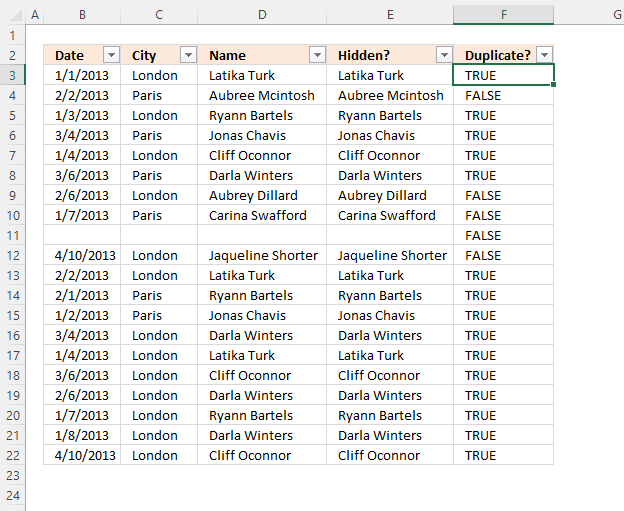Here is how to create an AutoFilter for the data set in cell range B2:F22.

1. Select any cell in cell range B2:F22.
2. Go to tab "Data" on the ribbon.
3. Press with left mouse button on the "Filter" button.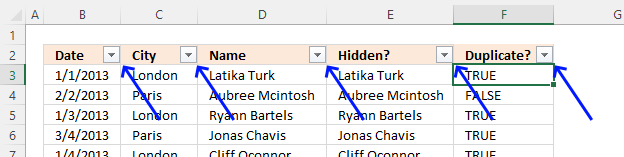Arrows in the column header appear, they let you apply filters. The next step shows you how.

### 3.4 Apply Filter criteria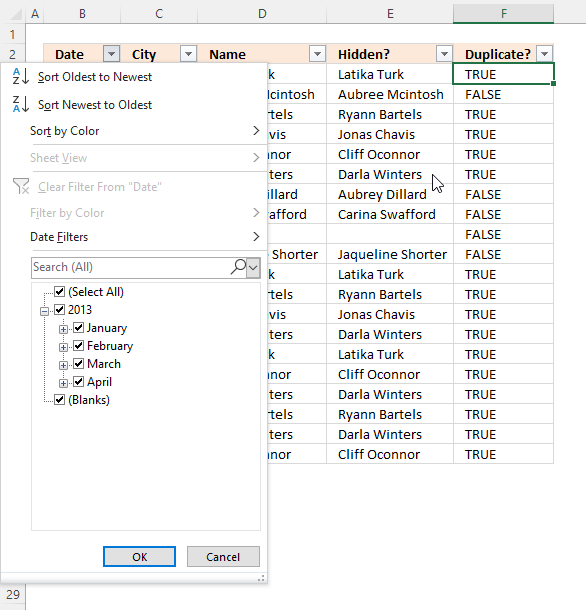1. Press with left mouse button on the arrow next to the column header "Date".
2. A popup menu shows up, deselect all checkboxes except "January".
3. Press with left mouse button on "OK" button.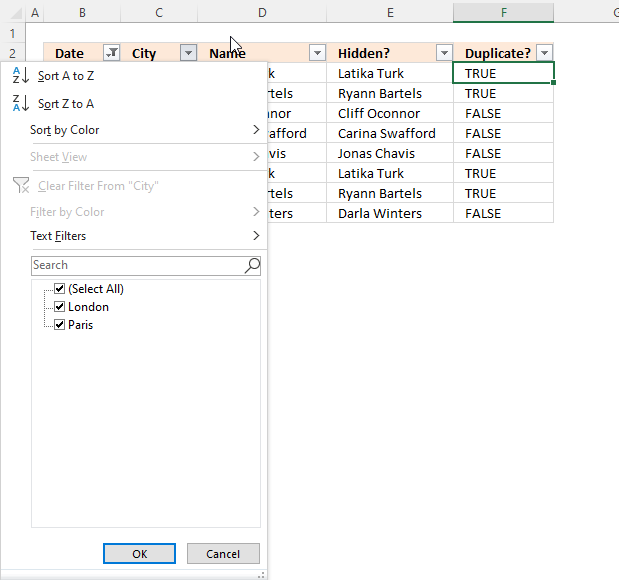1. Press with left mouse button on the arrow next to the column header "City".
2. A popup menu shows up, deselect all checkboxes except "London".
3. Press with left mouse button on the "OK" button.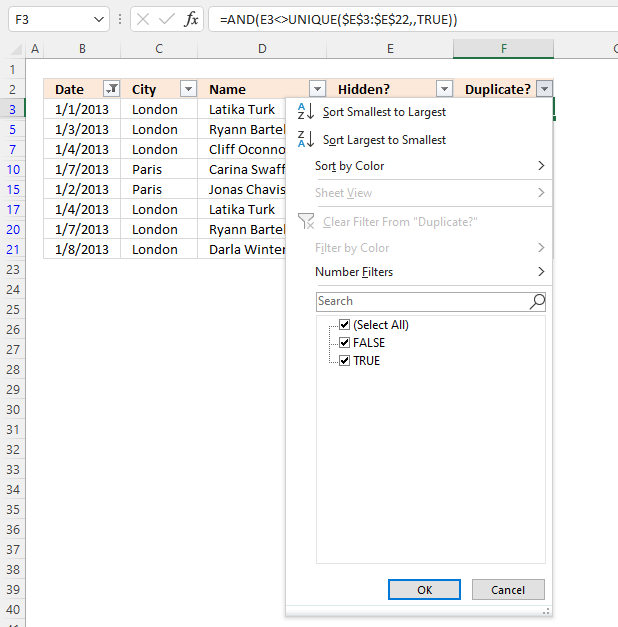1. Press with left mouse button on the arrow next to the column header "Duplicate?".
2. A popup menu shows up, deselect all checkboxes except "TRUE".
3. Press with left mouse button on the "OK" button.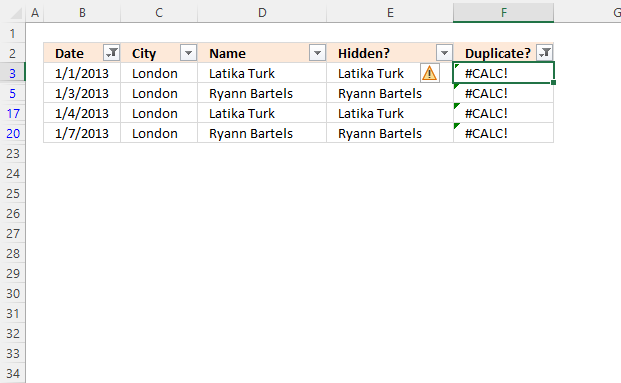Column E now shows all duplicate values.

## 4. Filter duplicate values using criteria - Excel Table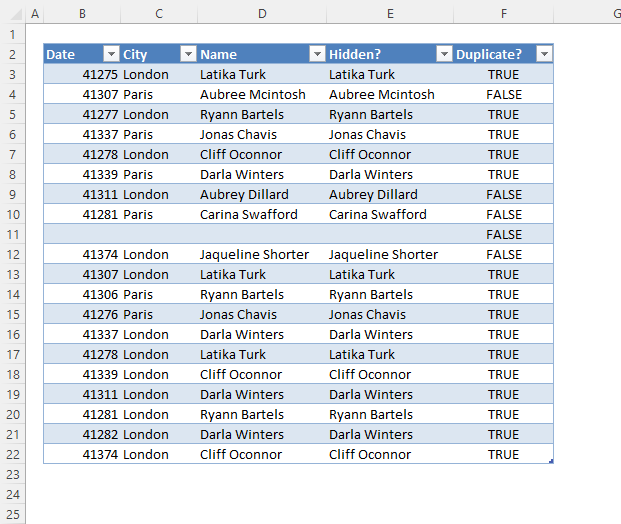This example shows how to filter duplicate values using an Excel Table. It is very similar to section 3, however, Excel fills the remaining cells below automatically after you have entered a formula.

Here is how to convert a cell range to an Excel Table.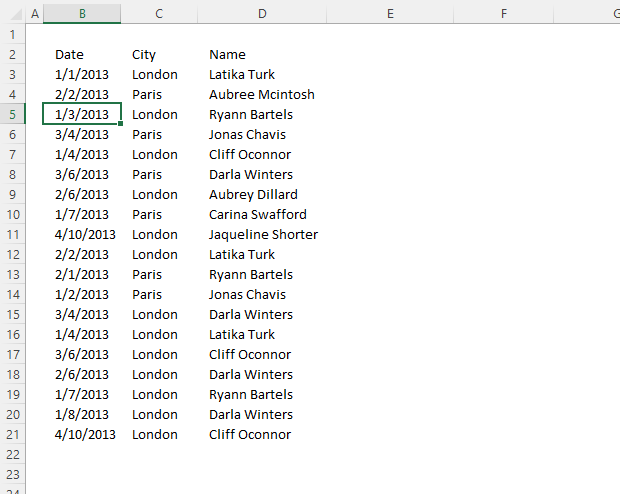1. Select any cell in the data set.
2. Go to tab "Insert" on the ribbon.
3. Press with left mouse button on the "Table" button.
4. A dialog box appears.
5. Press with left mouse button on the "OK" button.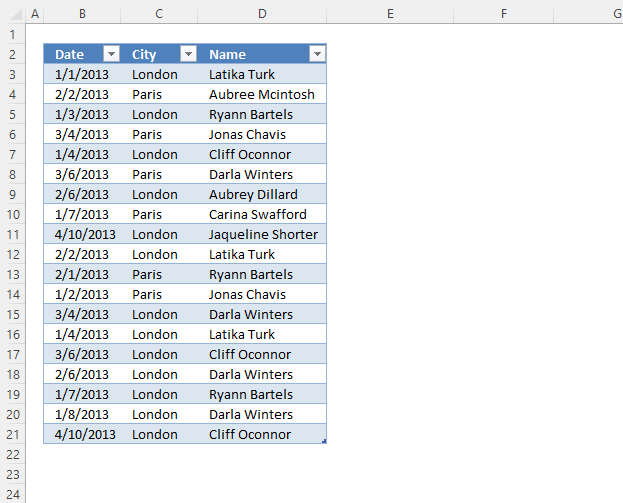### 4.1 Enter formulasSee section 3.1 and 3.2 above on how to enter the formulas.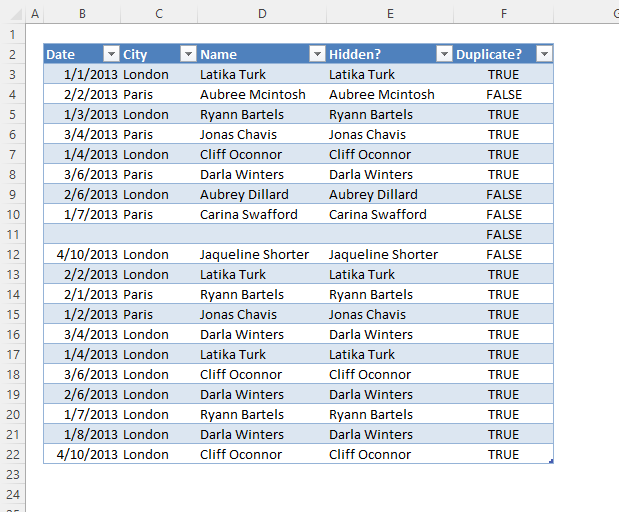### 4.2 Apply criteria to Excel TableRead section 3.4 above on how to filter the Excel Table. The technique is the same for both AutoFilter and the Excel Table.